TitleCollege Algebra
Tutorial 28: Parallel and Perpendicular LinesLearning Objectives

 After completing this tutorial, you should be able to: Find the slope of a line that is parallel to a given line. Find the slope of a line that is perpendicular to a given line.Introduction

 This tutorial looks at the relationship between the slopes of parallel lines as well as perpendicular lines.  Once again we are going to be using material from our math past to help find the new concept.  You will need to know how to find the slope of a line given an equation and how to write the equation of a line.  If you need a review on these concepts, feel free to go to Tutorial 26: Equations of Lines.    Let's see what you can do with parallel and perpendicular.Tutorial

 Parallel Lines and Their Slopes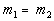In other words, the slopes of parallel lines are equal. Note that two lines are parallel if their slopes are equal and they have different y-intercepts.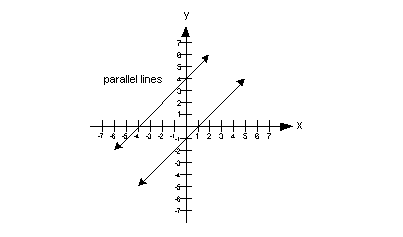Perpendicular Lines and Their Slopes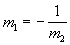In other words, perpendicular slopes are negative reciprocals of each other.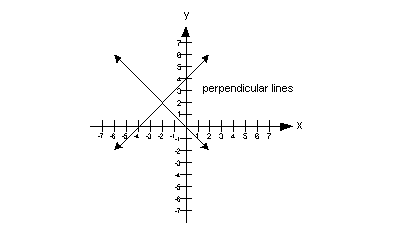Here is a quick review of the slope/intercept form of a line.  If you need more of a review on how to use this form, feel free to go to Tutorial 26: Equations of Lines.

 Slope/Intercept Equation of a LineIf your linear equation is written in this form, m represents the slope and b represents the y-intercept. This form can be handy if you need to find the slope of a line given the equation.Example 1:    Find the slope of any line that is a) parallel and b) perpendicular to the line.

 Before we tackle finding the parallel and perpendicular slopes it really can help us out if we find the slope of the given line. Recall that when you are given the equation of a line that you can find the slope of it by writing it in the slope/intercept form,, where m is the slope and b is the y-intercept of the line. This equation is already written in the slope/intercept form: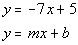*Written in slope/intercept form

 Lining up the form with the equation we have been given, can you see what the slope is? If you said -7, you are correct!!!   Slope of the parallel line: Since parallel lines have the same slope what do you think the slope of any parallel line to this line is going to be? Pat yourself on the back if you said -7. Slope of the perpendicular line: Since the slopes of perpendicular lines are negative reciprocals of each other, what do you think the slope of any perpendicular line to this line is?  Give yourself a high five if you said 1/7. Remember that you take the reciprocal which is -1/7 and then you negate it to get the 1/7 for your perpendicular slope.   The slope of any parallel line to the line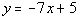is -7 and the slope of any perpendicular line to the lineis 1/7.Example 2:    Find the slope of the line that is a) parallel and b) perpendicular to the line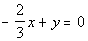.

 Before we tackle finding the parallel and perpendicular slopes it really can help us out if we find the slope of the given line. Recall that when you are given the equation of a line that you can find the slope of it by writing it in the slope/intercept form,, where m is the slope and b is the y-intercept of the line. Rewriting this equation in the slope/intercept form we get: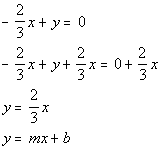*Inverse of sub. 2/3x is add. 2/3x   *Written in slope/intercept form

 Lining up the form with the equation we got, can you see what the slope is? If you said 2/3, you are correct!!!   Slope of the parallel line: Since parallel lines have the same slope what do you think the slope of the parallel line is going to be? Pat yourself on the back if you said 2/3. Slope of the perpendicular line: Since the slopes of perpendicular lines are negative reciprocals of each other, what do you think the slope of the perpendicular line is?  Give yourself a high five if you said -3/2. Remember that you take the reciprocal which is 3/2 and then you negate it to get the -3/2 for your perpendicular slope.   The slope of the parallel line is 2/3 and the slope of the perpendicular line is -3/2.Example 3:    Find the slope of the line that is a) parallel and b) perpendicular to the line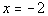.

 Do you remember what special type of line this equation is?  It is a vertical line.  If you need a review on vertical lines, feel free to go to Tutorial 27: Graphing Lines. What is the slope of a vertical line?  If you said undefined, you are right on.   Slope of the parallel line: Since parallel lines have the same slope, what do you think the slope of the parallel line is going to be? Pat yourself on the back if you said undefined.   Slope of the perpendicular line: Since slopes of perpendicular lines are negative reciprocals of each other, what do you think the slope of the perpendicular line is?  This one is a little trickier.  Vertical lines and horizontal lines are perpendicular to each other.  The slope of the perpendicular line in this case would be the slope of a horizontal line which would be 0.    The slope of the parallel line is undefined and the slope of the perpendicular line is 0.Example 4:    Find the slope of the line that is a) parallel and b) perpendicular to the line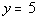.

 Do you remember what special type of line this equation is?  It is a horizontal line.  If you need a review on horizontal lines, feel free to go to Tutorial 27: Graphing Lines. What is the slope of a horizontal line?  If you said 0, you are right on.   Slope of the parallel line: Since parallel lines have the same slope, what do you think the slope of the parallel line is going to be? Pat yourself on the back if you said 0.   Slope of the perpendicular line: Since slopes of perpendicular lines are negative reciprocals of each other, what do you think the slope of the perpendicular line is?  This one is a little trickier.  Vertical lines and horizontal lines are perpendicular to each other.  The slope of the perpendicular line in this case would be the slope of a vertical line which would be undefined.    The slope of the parallel line is 0 and the slope of the perpendicular line is undefined.

 Here is a quick review of the point/slope form of a line.  If you need more of a review on how to use this form, feel free to go to Tutorial 26: Equations of Lines

 Point/Slope Form of an Equation A line going through the point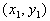and  having slope of m would have the equation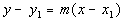We can use this form to plug into when we need to come up with a linear equation.  When writing an equation of a line, keep in mind that you ALWAYS need two pieces of information when you go to write an equation: ANY point on the line Slope Once you have these two pieces of information, you plug the x and y values from your point and the slope (m) value into the point/slope formula.Example 5:  Write an equation for the line in point/slope form and slope/intercept form that passes through (-2, -5) and parallel to the line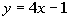.

 What are the two things we need to write an equation of a line???? If you said any point on the line and the slope, you are correct.   We have a point, however what about the slope?  Does this mean we can’t work out the problem?  You are not going to get off that easily.  We need to do a little digging to get our slope. As mentioned above, parallel lines have the same slope.  So, if we know the slope of the line parallel to our line, we have it made.   Let’s find the slope of the given line: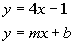*Slope/intercept form of the line

 Now keep in mind that this is not the equation of our line but of a line parallel to our line.  We needed to write it this way so we could get the slope.  And it looks like the slope is 4.  Since our line is parallel to a line that has a slope of 4, our line also has a slope of 4.  OK, now we have our slope, which is 4.  Now it is just like problems in Tutorial 26: Equations of Lines, we put the slope and one point into the point/slope equation. Point/Slope Form: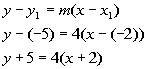*Point/slope form of the line

 Make sure that you are careful when one of your values is negative and you have to subtract it as we did in line 2.  y - (-5) is not he same as y - 5 and  x - (-2)  is not the same as x - 2.

 Next, we want to write it in the Slope/Intercept Form, which basically means we need to solve for y: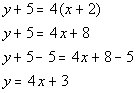*Dist. the 4 through (  ) *Inverse of add. 5 is sub. 5 *Slope/intercept form of the line

 The equation of the line that passes through (-2, -5) and is parallel to the lineis  y + 5 = 4(x + 2)   OR   y = 4x + 3.Example 6:  Write an equation for the line in point/slope form and slope/intercept form that passes through (3, 2) and perpendicular to the line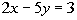.

 What are the two things we need to write an equation of a line???? If you said any point on the line and the slope, you are correct. We have a point, however what about the slope?  Does this mean we can’t work out the problem?  You are not going to get off that easily.  We need to do a little digging to get our slope. As mentioned above, the slopes of perpendicular lines are negative reciprocals of each other.  So, if we know the slope of a line perpendicular to our line, we have it made.   Let’s find the slope of the given line: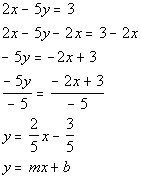*Inverse of add. 2x is sub. 2x   *Inverse of mult. by -5 is div. by -5 *Slope/intercept form of the line

 Now keep in mind that this is not the equation of our line but of the line parallel to our line.  We needed to write it this way so we could get the slope.  And it looks like the slope is 2/5.  Since our line is perpendicular to a line that has a slope of 2/5, our line has a slope of -5/2 (the negative reciprocal of 2/5). OK, now we have our slope, which is -5/2.  Now it is just like problems in Tutorial 26: Equations of Lines, we put the slope and one point into the point/slope equation. Point/Slope Form: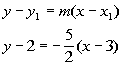*Point/slope form of the line

 Next, we want to write it in the Slope/Intercept Form, which basically means we need to solve for y: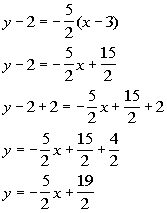*Dist. the -5/2 through (  )     *Inverse of sub. 2 is add. 2       *Slope/intercept form of the line

 The equation of the line that passes through (3, 2) and perpendicular to the lineis y - 2 = -5/2(x - 3)  OR   y = -5/2x + 19/2.Practice Problems

 These are practice problems to help bring you to the next level.  It will allow you to check and see if you have an understanding of these types of problems. Math works just like anything else, if you want to get good at it, then you need to practice it.  Even the best athletes and musicians had help along the way and lots of practice, practice, practice, to get good at their sport or instrument.  In fact there is no such thing as too much practice. To get the most out of these, you should work the problem out on your own and then check your answer by clicking on the link for the answer/discussion for that  problem.  At the link you will find the answer as well as any steps that went into finding that answer.Practice Problems 1a - 1c: Find the slope of the line that is a) parallel and b) perpendicular to the given line.

 1a.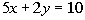(answer/discussion to 1a) 1b.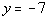(answer/discussion to 1b)

 1c.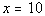(answer/discussion to 1c)Practice Problems 2a - 2b: Write an equation for the line in point/slope form and slope/intercept form that has the given condition.

 2a. Passes through (-7, 2) and is parallel to. (answer/discussion to 2a)

 2b. Passes through (3, -1) and is perpendicular to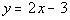(answer/discussion to 2b)Need Extra Help on these Topics?

The following are webpages that can assist you in the topics that were covered on this page:

 http://www.wtamu.edu/academic/anns/mps/math/mathlab/int_algebra/int_alg_tut15_slope.htm#parallel This webpage helps you with parallel and perpendicular lines.

Go to Get Help Outside the Classroom found in Tutorial 1: How to Succeed in a Math Class for some more suggestions.

Last revised on Feb. 20, 2010 by Kim Seward.